Get instant live expert help with Excel or Google Sheets“My Excelchat expert helped me in less than 20 minutes, saving me what would have been 5 hours of work!”

#### Post your problem and you’ll get Expert help in seconds.

Your message must be at least 40 characters
Our professional Expert are available now. Your privacy is guaranteed.

# Excel DATA VALIDATION Using the ISNUMBER and FIND Function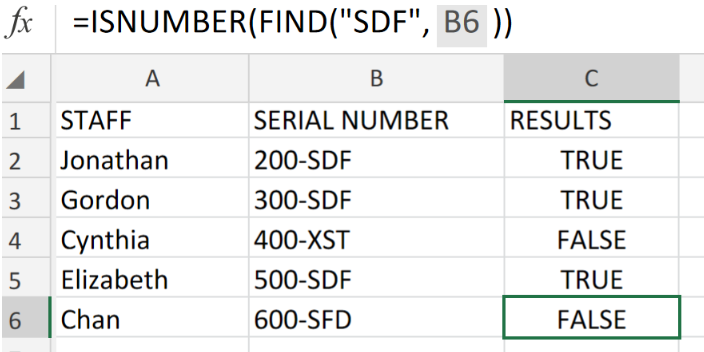Figure 1. Excel DATA VALIDATION Using the ISNUMBER and FIND Function.

If we want to permit entry of data values containing a specified text string only, we are going to make use of a custom data validation formula derived from the FIND and ISNUMBER functions in Excel.

## Formula Syntax

`=ISNUMBER(FIND("txt",A1))`

This formula will force users to only enter a specific text string into the cells of our worksheet.

## How to use the Excel DATA VALIDATION with the ISNUMBER and FIND Functions

By using this formula, the FIND function searches for the specified text string and if found, it identifies its location as a number then, based on this number given by the FIND function, the ISNUMBER function returns a TRUE result.

We can get this done in 3 simple steps;

1. Arrange the data available for validation and arrange in our worksheet.

See worksheet example  below;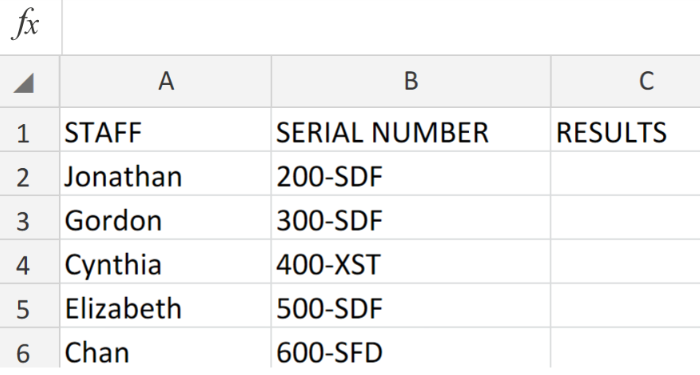Figure 2. Excel DATA VALIDATION Using the ISNUMBER and FIND Function.

Our purpose here is to search the cell range B2:B6 and identity the specific text “SDF”.

Be sure to provide a few empty cells for Excel to return the desired results.

1. In our worksheet example above, the Data Validation formula we will type into cell C2 is;

`=ISNUMBER(FIND("SDF",B2))`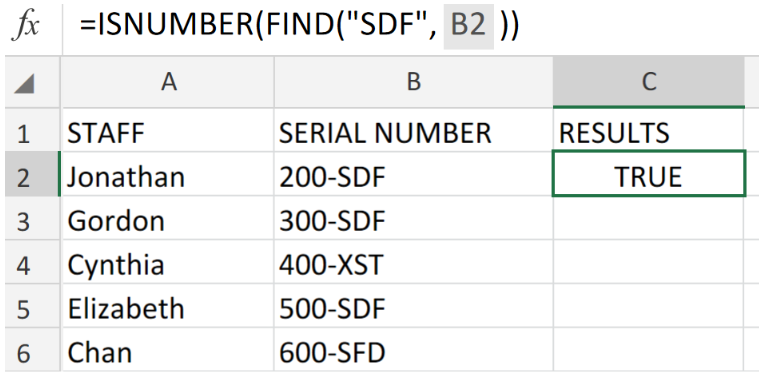Figure 3. Excel DATA VALIDATION Using the ISNUMBER and FIND Function.

Excel located our specific text string in cell B2 and returned a TRUE result.

1. Copy a modified version of the Data Validation formula down in the empty cells of the RESULTS column to get the other results.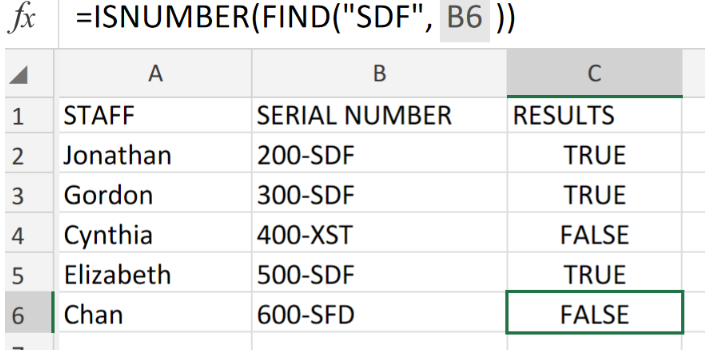Figure 4. Excel DATA VALIDATION Using the ISNUMBER and FIND Function.

Whenever a user adds or changes a cell value, data validation rules are triggered.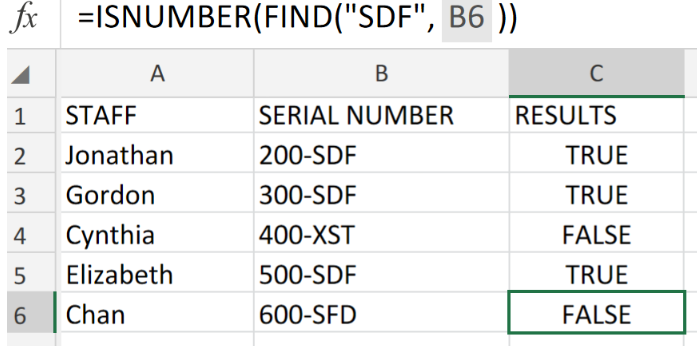Figure 5. Final Result.

## Instant Connection to an Expert through our Excelchat Service:

Our live Excelchat Service is here for you. We have Excel Experts available 24/7 to answer any Excel questions you may have. Guaranteed connection within 30 seconds and a customized solution for you within 20 minutes.

### Did this post not answer your question? Get a solution from connecting with the expert.Another blog reader asked this question today on Excelchat:
Related blogs## Subscribe to Excelchat.coAnother blog reader asked this question today on Excelchat: